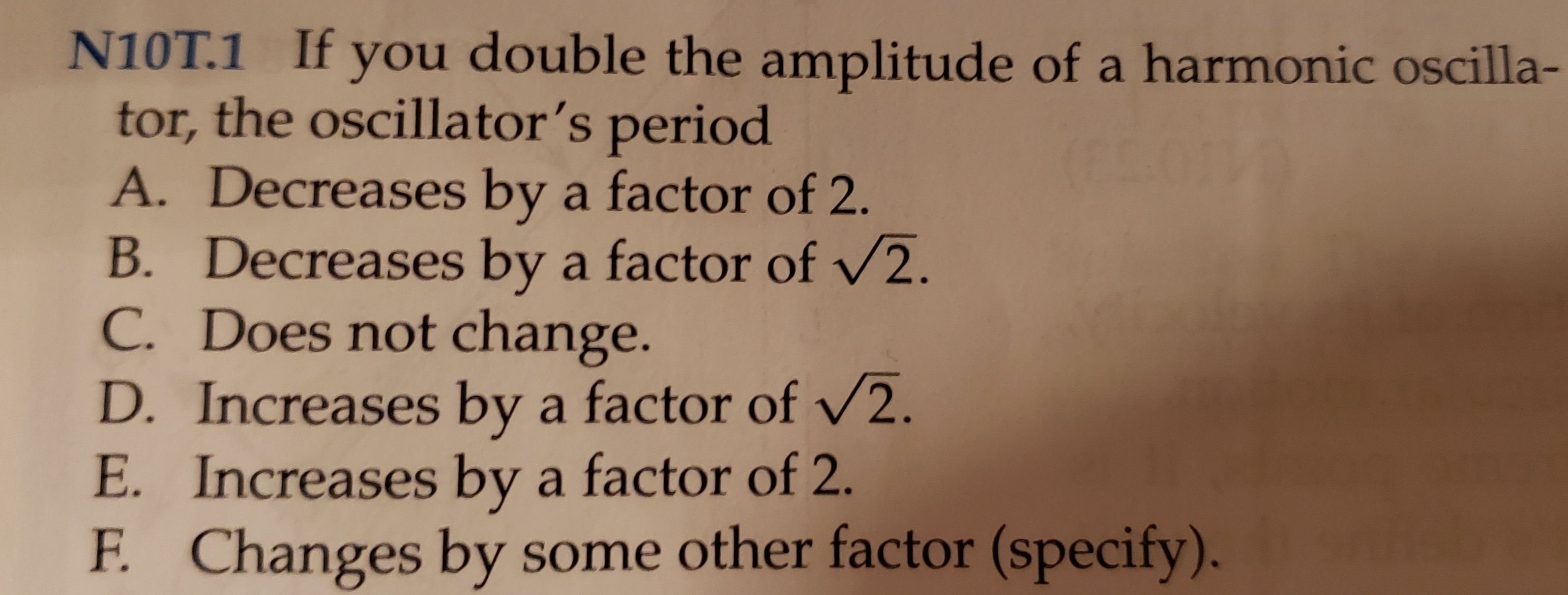# N10T.1 If you double the amplitude of a harmonic oscilla- tor, the oscillator's period A. Decreases by a factor of 2. B. Decreases by a factor of /2. C. Does not change. D.Increases by a factor of /2. E. Increases by a factor of 2. Changes by some other factor (specify). F.

Questionhelp_outlineImage TranscriptioncloseN10T.1 If you double the amplitude of a harmonic oscilla- tor, the oscillator's period A. Decreases by a factor of 2. B. Decreases by a factor of /2. C. Does not change. D.Increases by a factor of /2. E. Increases by a factor of 2. Changes by some other factor (specify). F. fullscreen

### Want to see this answer and more?

Experts are waiting 24/7 to provide step-by-step solutions in as fast as 30 minutes!*